John F. Gibson Center for Nonlinear Science Georgia Institute of Technology

## Movies of plane Couette flow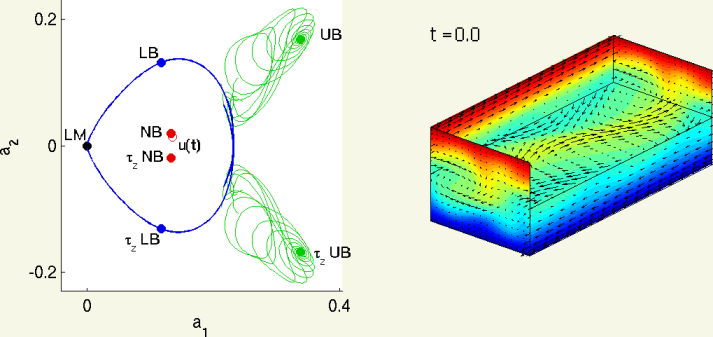An example: State-space on left, velocity field on right. See Dual views section for details.

See also: My research, in plain words and Note on video formats.

### What these movies illustrate

There are two kinds of animations here:
• 3D movies of velocity fields, which show turbulent flows visiting unstable coherent structures, and
• State-space trajectories, which show
• that the coherent structures in the 3D movies result from close passes to unstable equilibrium solutions of Navier-Stokes, and
• that these solutions and their unstable manifolds impart a rigid structure to state space, which organizes the turbulent dynamics.
The
Dual views section shows some of these animations side-by-side. In these, you can see coherent structures appear in the turbulent velocity field as the state-space trajectory makes close passes to equilibrium solutions of Navier-Stokes.

In the 3D velocity fields, the top wall moves toward viewer, and the bottom wall moves away with equal and opposite velocity. The velocity field is coded by color and 2D vectors. Red: fluid moving toward viewer. Blue: fluid moving away from the viewer. Arrows: fluid velocity transverse to the direction of wall motion. The top half of the fluid is cut away to show the midplane velocity field, halfway between the upper and lower walls.

### Plane Couette, from experimental to idealized flows

These animations show a sequence of simplifications, starting with a flow with close to experimental conditions and ending with a qualitatively similar flow whose dynamics are simpler to analyze.

1. Plane Couette turbulence in a periodic cell with large aspect ratio (2007-09-11)
explanation of setup and color coding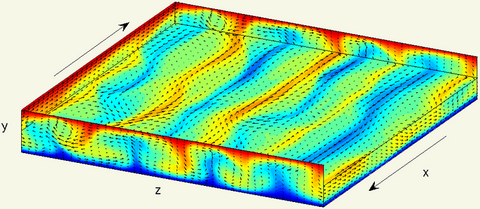In both cases the initial velocity field is small, smooth, and incompressible. meets the boundary conditions, and satisfies none of the plane Couette symmetries. The periodic orbit is 3 x 4 copies of the P35 orbit, slightly stretched and scaled to fit [Lx, Ly, Lz] = [15, 2, 15]. The Reynolds number is 400, the spatial grid is 96 x 33 x 128, and the time step is dt = 0.03125.

What is striking about these simulations is that all non-laminar initial conditions appear to settle into the similar patterns of behavior, consisting of episodes of highly ordered motion among streamwise counter-rotating rolls, interspersed by periods of less ordered, more turbulent motions.

This observation motivates the empirical search for small aspect ratios large enough to accommodate a pair of unstable counter-rotating rolls, following Hamilton, Kim and Waleffe.

2. Plane Couette turbulence in a small periodic cell.
The flow domain here is the "HKW" cell, Lx = 7π/4 = 5.50, Lz = 6π/5 = 3.76, found empirically by Hamilton, Kim and Waleffe to be the smallest that appears to sustain turbulence. The initial condition is a random perturbation from laminar flow (2007-09-04)

3. "Symmetric turbulence," within an invariant symmetric subspace of the HKW cell. (2007-09-03)
The initial condition is a field from the previous simulation, translated streamwise, spanwise to optimize the s1,s2 symmetries (to within 2% in the energy norm), and then projected to lie exactly in the symmetric subspace. The s1,s2 symmetries are
s1 : [u,v,w](x,y,z) -> [u,v,-w](x+Lx/2, y, z)
s2 : [u,v,w](x,y,z) -> [-u,-v,w](-x+Lx/2, -y, z+Lz/2)

The symmetric subspace is space of velocity fields left invariant under both s1 and s2.

All the exact invariant solutions (equilibria and their stable/unstable manifolds, periodic orbits) shown in what follows belong to this symmetric subspace. What is surprising about the above simulations is that --even though a typical initial state of a fluid has a zero probability of being within (or entering) the symmetric subspace-- the steady state turbulent dynamics seems to stays close to it for all times. This observation motivates what follows, a closer investigation of the geometry of the state-space flow within the symmetric subspace.

### Dual views of velocity field and state-space evolution

The following movies show a plane Couette velocity field evolving under Navier-Stokes on the right and the corresponding state-space trajectory on the left. The evolution of the field is computed with a CFD algorithm. The flow and simulation parameters are [Lx, Ly, Lz] = [5.51, 2, 2.51], Re = 400, 32 x 49 x 32, and dt = 0.03125. The fields in this section are within the s1,s2 invariant subspace defined above.The state-space projection is from the 10^5 dimensional space of free variables in the CFD algorithm onto a 2d plane e1,e2 formed from linear combinations of the upper-branch equilibrium and its half-cell translations, with the laminar equilibrium as the origin. For example, e1 = (1 + τx + τz + τxz) uUB, normalized to ||e1|| = 1, where τx is a translation operator that shifts a velocity field by half the cell length in x. The norm and projection operator are defined by the L2 inner product. For example a1(t) = 1/||V|| ∫V u(x,t) ∙ e1(x) dx, where V is the cell volume.

Why does this make sense? See the Gibson, Halcrow and Cvitanović paper.

The labeled points are
 LM LB UB NB u(t): Laminar equilibrium Lower-branch equilibrium(Nagata, Waleffe) Upper-branch equilibrium (Nagata, Waleffe) "Newbie" equilibrium (Gibson, Halcrow, Cvitanović) the time-varying velocity field, evolving under Navier-Stokes

1. Transient turbulence from the unstable manifold of the NB equilibrium.
Other encodings:

Legend: The state-space trajectory is plotted against three exact equilibria of plane Couette flow: the lower-branch (LB, blue), the upper-branch (UB, green), and the "newbie" (NB, red) equilibria, plus the laminar solution (LM, black) (see Equilibria). The half-cell translations in x and z of the equilibria are points indicated with prefix "τx", "τz", and "τxz". The blue and green solid lines show the 1 and 2-dimensional unstable manifolds of the LB and UB equilibria. The moving red dot is the state of the fluid velocity field evolving under the Navier-Stokes equations.

Partial narrative:

• t=0-150 : The initial condition is a small perturbation in the unstable manifold of the NB equilibrium. The perturbation is small enough that no visible changes occur until about t=150.
• t=150-280 : An oscillation around the NB equilibrium, governed by the NB's unstable complex eigenvalue.
• t=280-300 : A close pass to the half-cell z-translated LB equilibrium, escape back toward turbulence along LB's 1d unstable manifold.
• t=300-1200 : Close passes to UB unstable manifold, equilibria still waiting to be discovered, z-shift LB, etc.
• t=1200-1350 : Decay to laminar flow.

2. Transient turbulence from the unstable manifold of the UB equilibrium. (12 MB)
This movie shows several close passes the lower-branch equilibrium.

Equilibria teach us a lot about dynamics, but as they are stationary, no turbulence takes place there. As the following dual-view movies show, the time dependence of typical unstable structures seen in turbulence is better captured by unstable periodic orbits.

Periodic orbits in symmetric subspace of narrow box
1. T=35.86 periodic orbit (1.3 MB)
This is a relative periodic orbit: the field returns to a streamwise half-cell translation in x of its original state after one period (T=35.86), and then to its original state after two periods (T=71.72).

2. T=97.08 periodic orbit (1.3 MB)
The P97 orbit is a true periodic orbit close to a two-period sequence of P35 orbits, with an extra wiggle.
Periodic orbits in symmetric subspace of HKW cell
1. My current collection of periodic orbits in the "HKW" box, Lx=1.75 pi, Lz=1.2pi. The P41p36 was calculated by Divakar Viswanath, and it appears to be the same as Kawahara and Kida's "gentle" orbit. P85p27 orbits was calculated by Viswanath and independently by J. Halcrow, P. Cvitanovic, and myself. Data for these orbits can be found in the channeflow database. All these orbits were computed with Viswanath's Newton-hookstep-GMRES search algorithm from close recurrences in a single u(x,t) trajectory. Each is a true periodic orbit in the s1,s2 symmetric subspace (no translations).

P19p02, P41p36, P46p23, P75p35, P76p82, P76p85, P85p27, P87p89, P88p90, P121p4

2. A turbulent trajectory making a close pass to the P68p07 orbit.### Equilibria and their stability

These plots show equilibria (steady states) of plane Couette flow for [Lx, Ly, Lz] = [5.51, 2, 2.51] and Re=400 computed with Viswanath's Newton-hookstep-GMRES search algorithm. Discretization is on a 32 x 35 x 32 dealiased grid. Truncated spectral coefficients are on the order 1e-6. Each field is a fixed point of the CFD algorithm to about the same accuracy.
• uLM is the classic laminar equilibrium --a linear profile (u,v,w) = (y,0,0).
• uLB,UB are the Nagata lower and upper branch equilibria (computed also by Waleffe, and refined here with Viswanath's algorithm).
• uNB, uNB2, and uEQ5 are new equilibria found by Gibson, Halcrow, and Cvitanović.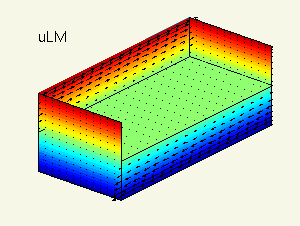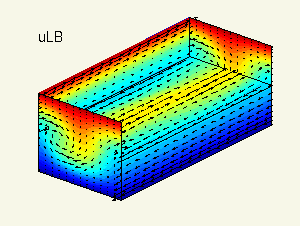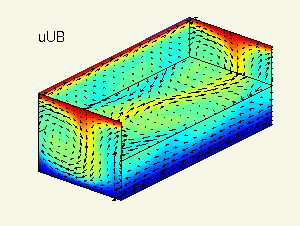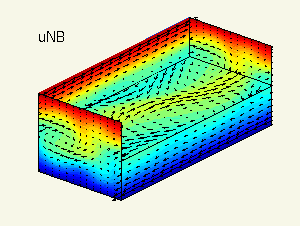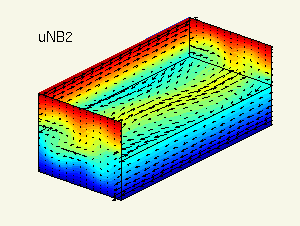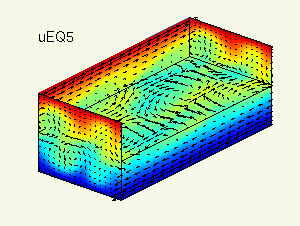Here are the eigenvalues of the above equilibria, computed with Arnoldi iteration. The left plot shows the complete set of eigenvalues; on the right are the eienvalues in the s1,s2-symmetric subspace. Accuracy is about 1e-7.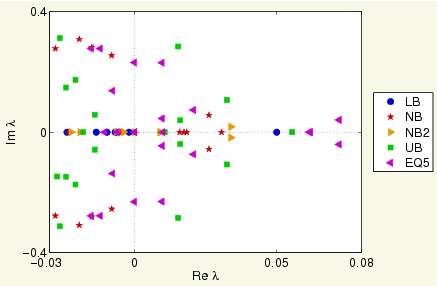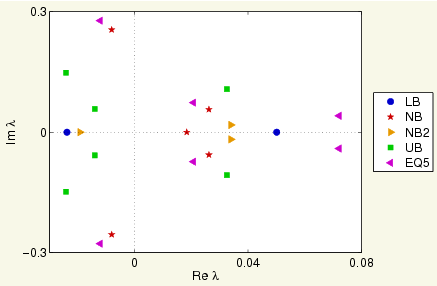### Streak instabilities and the self-sustaining process

The following movies illustrate pieces of the self-sustaining process theory presented in Waleffe Phys Fluids 1997. Please refer to that paper for details. The movies show the growth of streamwise-varying instabilities of streamwise-constant streaks. Under the linearized dynamics with the streaks held constant, the instabilities evolve as u(t) = u0 + ε eμ t (vr cosωt - vi sinωt) where λ = μ + i ω is the complex eigenvalue and vr and vi are real-valued superpositions of the complex eigenfunction.
1. An unstable eigenfunction of frozen streaks (2.2 MB) does not show dynamics, rather shows real-valued superpositions u0 + ε (vr cos&theta - vi sin&theta) for varying θ, which goes from 0 to 2 π over the course of the movie.
2. Linear dynamics around frozen roll-streak (1.8 MB) shows the initial condition u0 + ε vr evolving as u(t) = u0 + ε eμ t (vr cosωt - vi sinωt) under the linearized dynamics. &epsilon |vr| = 0.01 |u0|.
3. DNS of streak instability (6.9 MB) shows the same initial condition u0 + ε vr evolving under the Navier-Stokes equations, computed with DNS.
4. DNS of streak instability, slightly different initial condition (5.3 MB) shows a similar movie to the one above, but starting with slightly weaker streaks and a slightly larger eigenfunction perturbation. If you have a little traiing in nonlinear dynamics you can probably can see where I'm going with this...
These are preliminary research results intended to whip up a frenzy of enthusiasm among my colleagues. Details will follow in publications. (2008-02-07)

### Channelflow.org

All computations were performed with CFD software from www.channelflow.org. Channelflow provides well-tested and easy-to-use implementations of a spectral CFD algorithm, Viswanath's algorithms for computing equilibria, traveling waves, periodic orbits, and linear stability modes, and a number of utilities for manipulating velocity fields and producing visualizations.

The animations were produced as uncompressed AVI files in with custom Matlab visualization scripts and then compressed and packaged as mp4s with the "mencoder" and "mp4creator" video utilities in Linux. The simulation software and scripts for producing movies can be downloaded from www.channelflow.org/download.

### References

John F. Gibson, in collaboration with: Jonathan Halcrow, Predrag Cvitanović, Fabian Waleffe, and Divakar Viswanath.